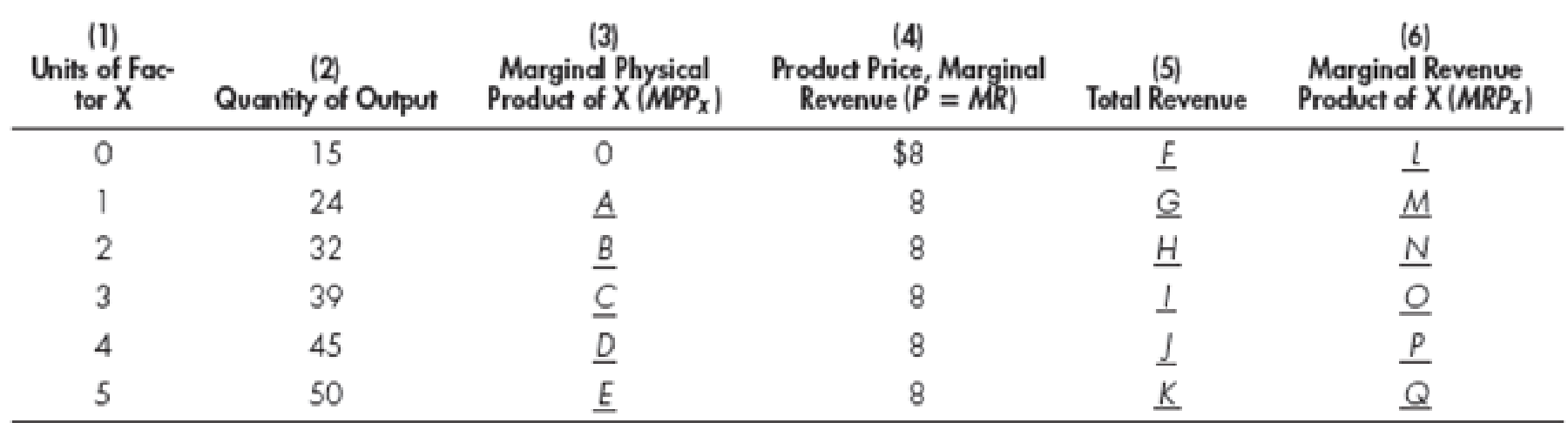Chapter 13, Problem 2WNG

Chapter
Section
Textbook Problem

On the basis of the preceding table, if the price of a factor is constant at \$48, how many units of the factor will the firm buy?To determine

Calculate the unit of factor that a firm buys at price \$48.

Explanation

A firm should purchase labor when the additional revenue earned by a firm using an additional unit of labor equals the cost incurred when additional unit of labor purchased...

Still sussing out bartleby?

Check out a sample textbook solution.

See a sample solution

The Solution to Your Study Problems

Bartleby provides explanations to thousands of textbook problems written by our experts, many with advanced degrees!

Get Started